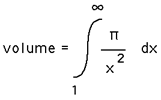or

# Paint bucket that can't be painted

Written by Paul Bourke
June 1999

Quite often one comes across seemingly mysterious objects in fractal geometry that have, say, zero volume and infinite surface area (see the menger sponge). There are however non intuitive results from more classical calculus and geometry, here's an example....

Consider the curve y = f(x) = 1/x from x=1 to infinity. Now rotate this curve into the z axes, around the x axis to form a curved cone-like object. A plane perpendicular to the x axis that cuts this surface of revolution is a circle with a radius of 1/x. The circumference of this circle is 2pi/x and the area of this circle is pi/x2.

This is sometimes known as Gabriel's horn or Gabriel's Trumpet.The volume is the integral of pi/x2 with respect to x evaluated between 1 and infinity, this is finite, namely pi.The surface area of our curved cone is infinite.We are left with the inescapable conclusion that our curved cone can hold finite amount of paint (the volume is finite) but we could never paint the outside surface (the surface area is infinite).